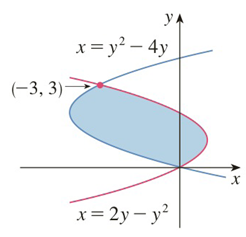Chapter 5.1, Problem 4E

Chapter
Section
Textbook Problem

# Find the area of the shaded region.To determine

To find:

The area of the shaded region

Explanation

1) Concept:

The area A of the region bounded by the curves x=f(y), x=g(y) and the lines y=a and y=b, where f and g are continuous and f(y) g(y) for all y in a, b is

A= abfy-gydy

2) Given:

3) Calculation:

From the given graph,

we have that x=2y-y2 is the right boundary curve and x=y2-4y   is the left boundary curve.

Let,  fy=2y-y2 and gy=y2-4y

The shaded region lies between y=0 to y=3

Formula-

Area  A= abfy

### Still sussing out bartleby?

Check out a sample textbook solution.

See a sample solution

#### The Solution to Your Study Problems

Bartleby provides explanations to thousands of textbook problems written by our experts, many with advanced degrees!

Get Started

#### Find f. f(x) = 5x2/3, f(8) = 21

Single Variable Calculus: Early Transcendentals, Volume I

#### Factoring Trinomials Factor the trinomial. 73. 3x2 16x + 5

Precalculus: Mathematics for Calculus (Standalone Book)

#### In Exercises 69-74, rationalize the numerator. 73. x2z3y

Applied Calculus for the Managerial, Life, and Social Sciences: A Brief Approach

#### Simplify: 4860

Elementary Technical Mathematics

#### True or False: is a convergent series.

Study Guide for Stewart's Multivariable Calculus, 8th

#### Sometimes, Always, or Never: The antiderivative of an elementary function is elementary.

Study Guide for Stewart's Single Variable Calculus: Early Transcendentals, 8th